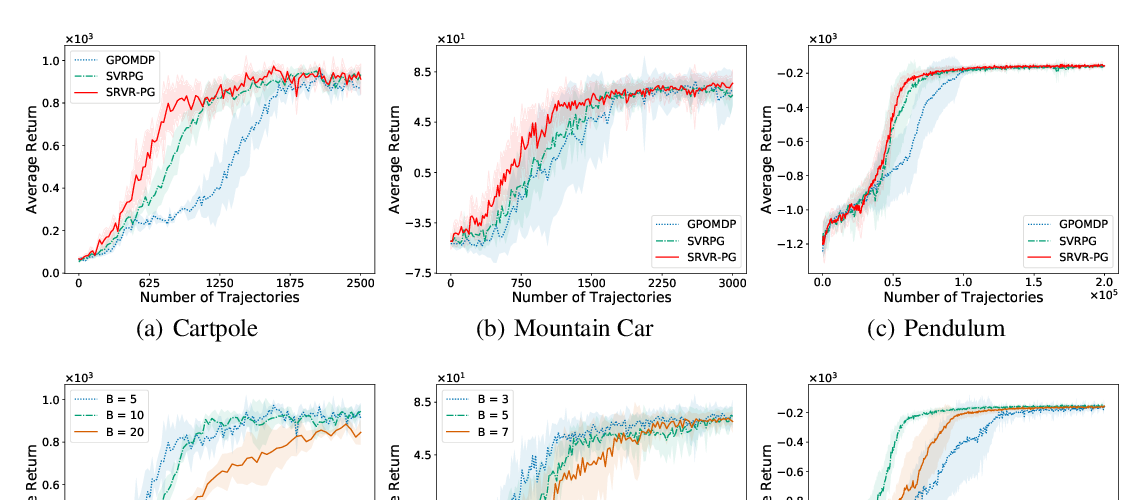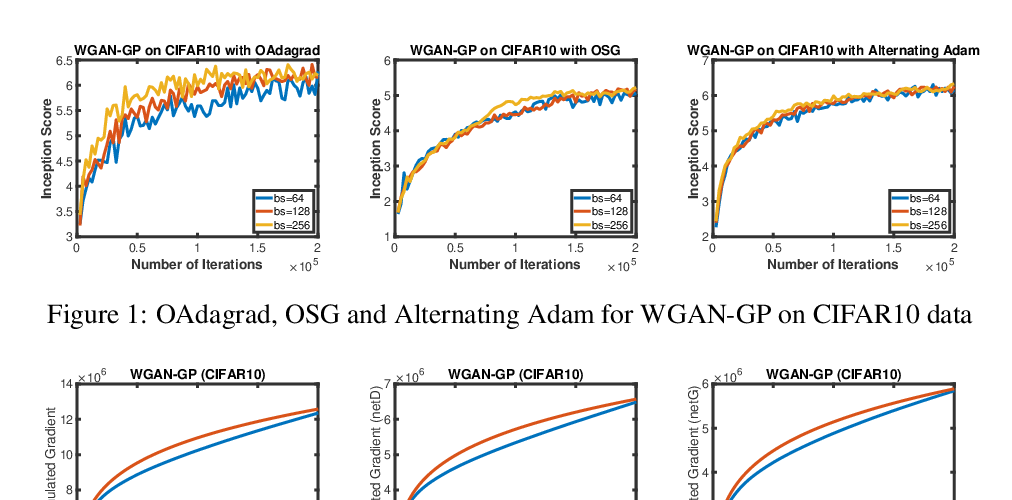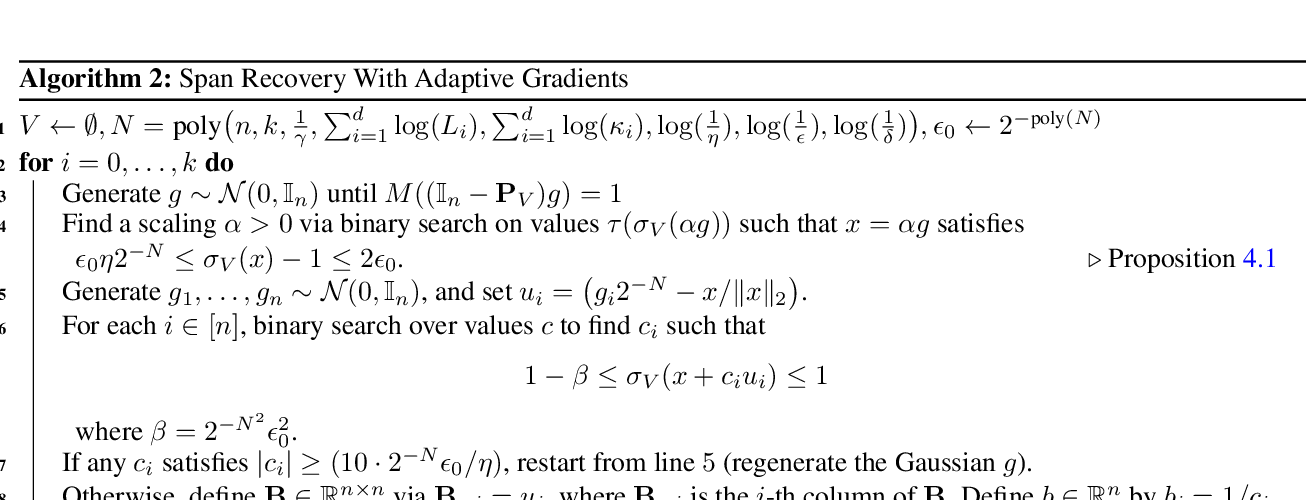## Polylogarithmic width suffices for gradient descent to achieve arbitrarily small test error with shallow ReLU networks

### Ziwei Ji, Matus Telgarsky

Abstract: Recent theoretical work has guaranteed that overparameterized networks trained by gradient descent achieve arbitrarily low training error, and sometimes even low test error. The required width, however, is always polynomial in at least one of the sample size $n$, the (inverse) target error $1/\epsilon$, and the (inverse) failure probability $1/\delta$. This work shows that $\widetilde{\Theta}(1/\epsilon)$ iterations of gradient descent with $\widetilde{\Omega}(1/\epsilon^2)$ training examples on two-layer ReLU networks of any width exceeding $\textrm{polylog}(n,1/\epsilon,1/\delta)$ suffice to achieve a test misclassification error of $\epsilon$. We also prove that stochastic gradient descent can achieve $\epsilon$ test error with polylogarithmic width and $\widetilde{\Theta}(1/\epsilon)$ samples. The analysis relies upon the separation margin of the limiting kernel, which is guaranteed positive, can distinguish between true labels and random labels, and can give a tight sample-complexity analysis in the infinite-width setting.

## Similar Papers

##### Sample Efficient Policy Gradient Methods with Recursive Variance Reduction
###### Pan Xu, Felicia Gao, Quanquan Gu,##### Towards Better Understanding of Adaptive Gradient Algorithms in Generative Adversarial Nets
###### Mingrui Liu, Youssef Mroueh, Jerret Ross, Wei Zhang, Xiaodong Cui, Payel Das, Tianbao Yang,##### Span Recovery for Deep Neural Networks with Applications to Input Obfuscation
###### Rajesh Jayaram, David P. Woodruff, Qiuyi Zhang,